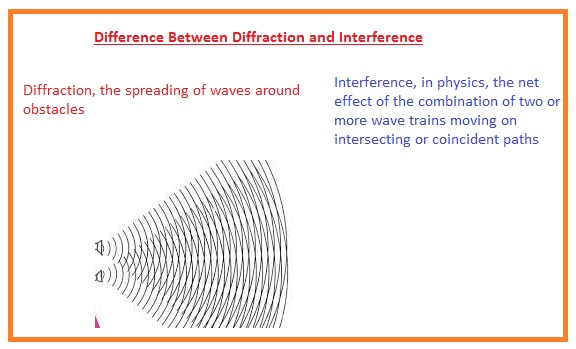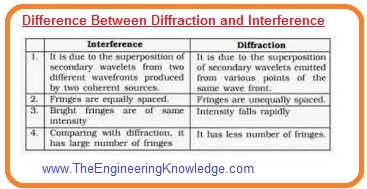In today’s tutorial, we will discuss the Difference Between Diffraction and Interference. The basic difference among diffraction and interference is that diffraction occurs when secondary wavelets generated by the numerous portion of wavefront superimpose with each other. While interference The basic difference among diffraction and interference is that diffraction occurs when secondary wavelets generated by the numerous portion of wavefront superimpose with each other. While interference occurs when light waves coming from two coherent light sources superimpose with each other.

The other difference is that the intensity of bright fringes is the same for interference but not for diffraction. In today’s post we will have a detailed look at both interference and diffraction and find their differences. So let’s get started with Difference Between Diffraction and Interference.

#### Difference Between Diffraction and Interference

Diffraction

• It occurs when moving waves get involemtn to the hinderence exsiting in the path of wave.
• It also define that wave bends when move across the body. This phenomen first time was disucsed in in 1660
• This process occur normally when the wave coming from such source htat is coherent hass single wavelength.
• It also works for such system where medium has variable refractive index.
• The dvd shoes diiffent colrs it is due to diffraction.
• Mirage is also example of diffraction.
• It used in hologram process
• This phenomenon occurs when the secondary wavelets superimpose with each other.
• For this process, the intensity of fringes is not similar for all fringes.• The direction of propagating waves changes when diffraction occurs.
• The width of the fringes is not equal.
• The space between the fringes is not uniform.
• To observe this phenomenon there is a need for obstacles or slit.
• For this process, there is less contrast between maxima and minima.

Interference

• It is the process in which two different waves mix in such a way that gives a wave of another type having some greater or less amplitude to the previous one.
• If waves are producing from a similar medium then constructive interference occurs.
• It used in different categories of waves like sounds, light radio. Etc.
• The resultant image form from the interface is known as an interferogram.
• For this process, there is no need for an obstacle.
• For this process, the direction propagating wave does not vary when the superimposition occurs.
• The contrast between maxima and minima is good.
• This process occurs when the light waves coming out from two different coherent light sources superimpose.
• Fringe intensity is similar for all fringes.
• The width of the fringes is equal.
• The space between fringes is uniform• According to this interference is divided into the 2 types.
• Constructive Interference
• Destructive Interference

Constructive Interference

• Constructive interference occurs when the 2 waves having a similar amplitude and phase superimpose with each other and their resultant wave amplitude is larger than the superimposing waves.
• In simple words, the resultant wave will have amplitude two times the amplitude of superimposing waves.

Destructive Interference

• Destructive Interference occurs when the 2 waves of having similar amplitude and opposite phase then their resultant wave have less amplitude than other 2 superimposing waves.
• When the 2 waves meet they will sum up and cancel each other due to the opposite phase angle.

That is a detailed post about the difference between diffraction and interference. If you have any query ask in comments. Thanks for reading. have a good day.

# Free SMT Assembly Monthly

New arrival for Aluminum boards, only \$2

## New users enjoy \$54 free coupons after signing up at JLCPCB successfully

\$54 coupons can also apply to 3D printing orders, for 3D printing special offer, it gets started at \$1